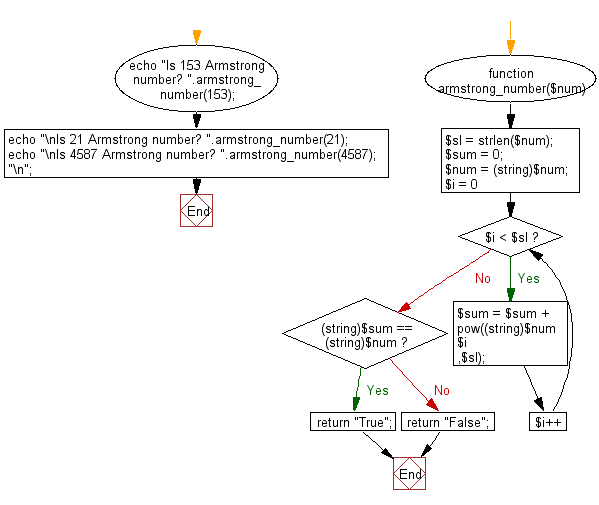﻿ PHP Exercise: Check if a number is an Armstrong number or not - w3resource# PHP Exercises: Check if a number is an Armstrong number or not

## PHP: Exercise-32 with Solution

Write a PHP program to check if a number is an Armstrong number or not. Return true if the number is Armstrong otherwise return false.

An Armstrong number of three digits is an integer so that the sum of the cubes of its digits is equal to the number itself. For example, 153 is an Armstrong number since 1**3 + 5**3 + 3**3 = 153

Sample Solution: -

PHP Code:

``````<?php
function armstrong_number(\$num) {
\$sl = strlen(\$num);
\$sum = 0;
\$num = (string)\$num;
for (\$i = 0; \$i < \$sl; \$i++) {
\$sum = \$sum + pow((string)\$num{\$i},\$sl);
}
if ((string)\$sum == (string)\$num) {
return "True";
} else {
return "False";
}
}
echo "Is 153 Armstrong number? ".armstrong_number(153);
echo "\nIs 21 Armstrong number? ".armstrong_number(21);
echo "\nIs 4587 Armstrong number? ".armstrong_number(4587);"\n";
?>
```
```

Sample Output:

```Is 153 Armstrong number? True
Is 21 Armstrong number? False
Is 4587 Armstrong number? False
```

Flowchart:PHP Code Editor:

Have another way to solve this solution? Contribute your code (and comments) through Disqus.

What is the difficulty level of this exercise?

﻿

New Content: Composer: Dependency manager for PHP, R Programming